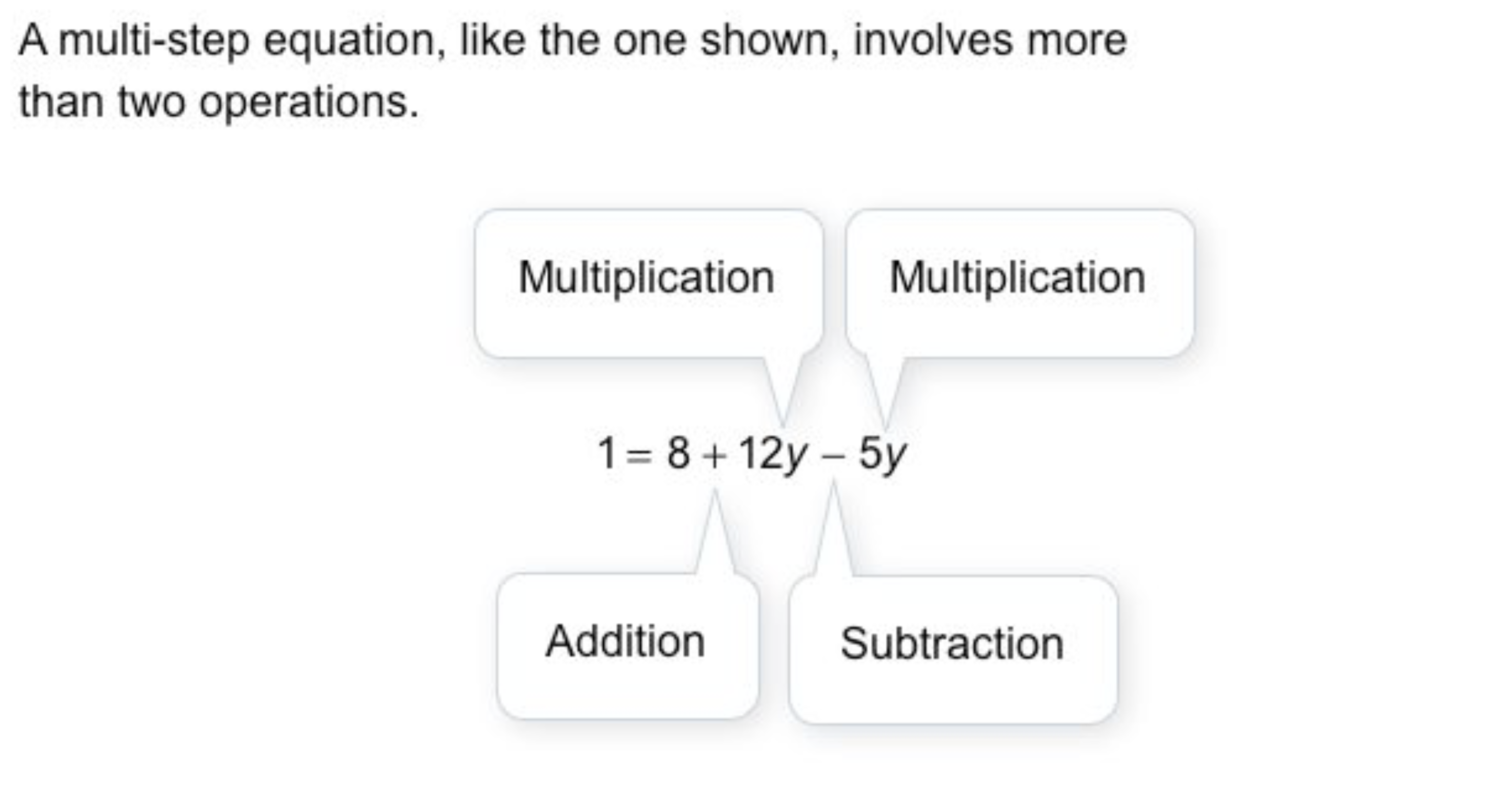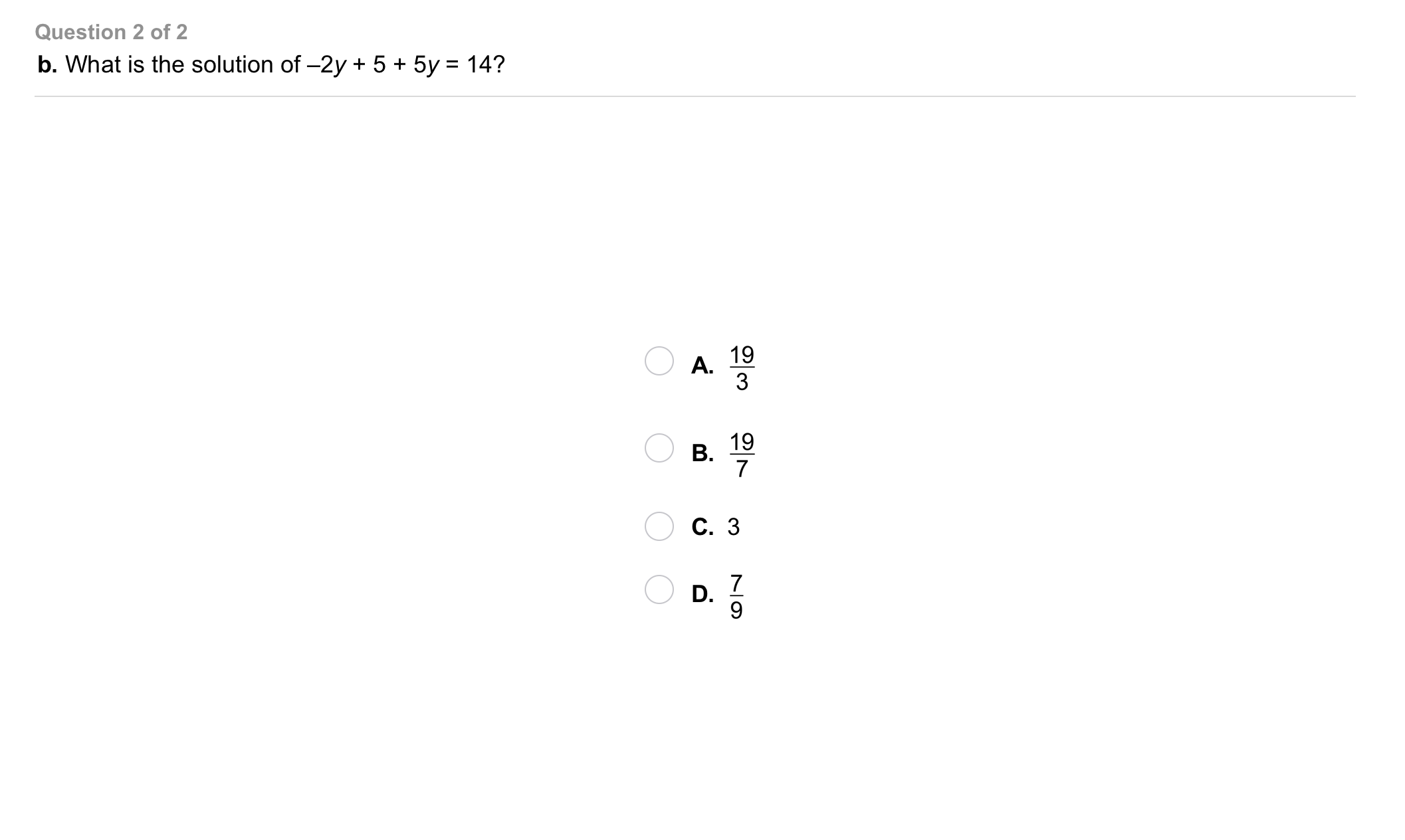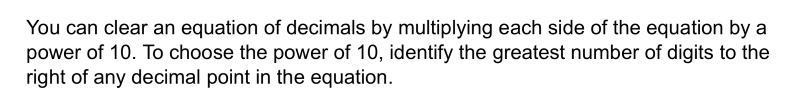Algebra 1 2-3 Guided Practice: Solving Multi-Step Equations
starstarstarstarstarstarstarstarstarstar
by Matthew Richardson
| 12 Questions1
1
10
Solve It! You are buying movie tickets online for a group of your friends. You have to enter the number of tickets you want on the screen shown. You are using a debit card to pay for the tickets and have a total of $45 to spend. How many tickets can you buy? Enter only a number.a b 2 Take Note: Recall that PEMDAS is an acronym to help you remember the correct order in which to "do" math operations. Take Note: Recall that when you solve an equation, you are essentially "un-doing" math operations to isolate variables. For this reason, you use the order of operations in reverse, SADMEP. 2 10 Take Note: Order the operations below based on SADMEP. 1. exponents 2. subtraction/addition 3. parentheses & other grouping symbols 4. division/multiplication3 3 10 Problem 1 Got It? A B C D4 4 10 Problem 1 Got It? A B C D5 5 10 Problem 2 Got It? Noah and Kate are shopping for new guitar stings in a music store. Noah buys 2 packs of strings. Kate buys 2 packs of strings and a music book. The book costs$16. Their total cost is \$72. How much is one pack of strings?
A
B
C
D
6
10
Enter only a number.
7
5
Problem 3 Got It? You probably used the Distributive Property to solve the equation in the item above. Could you have used the Division Property of Equality instead? In other words, could you have divided both sides of the equation by the same value to start to isolate the variable?
No
Yes8
b
9
8
10
Take Note: Since you will be working with fractions, it is appropriate to review the concepts of least common multiple and least common denominator.

A least common multiple [LCM] is the smallest multiple that a specific group of numbers has in common.

For example, 4, 6, and 12 have a LCM of 12 since 12 is the smallest multiple that 4, 6, and 12 all "go into."

A least common denominator [LCD] is the smallest multiple that a specific group of fractions' denominators have in common.

What is the LCD of the fractions below? Enter only a number.

9
10
Take Note: Remember that it is possible to completely clear fractions in many equations. To do so, multiply every term in the equation by the least common multiple of the denominators.

Consider the following equation:
\frac{2}{3}x+1=\frac{1}{2}y
By what number should you multiply all of the terms in the equation by to create an equivalent equation with no fractions? Enter only the number.

Hint: the resulting equivalent equation is:
4x+6=3y
Take Note: When solving an equation that contains fractions it is often helpful to establish a common denominator or to clear the fractions completely.
10
20
Problem 4 Got It? Show all of your work as you solve the equation.11
11
10
Problem 5 Got It?
A
B
C
D12
12
10
Take Note: Summarize the mathematical content of this lesson. What topics, ideas, and vocabulary were introduced?